# Class 10 Maths Worksheet for Application of Trigonometry

Given below are the Class 10 Maths Worksheet for Application of Trigonometry
(a)Match the column
(b)Multiple Choice Questions

## Match the Column

Question 1.
Match the Following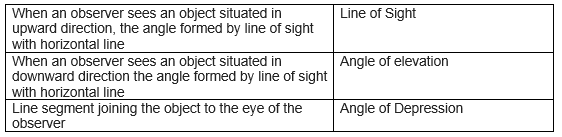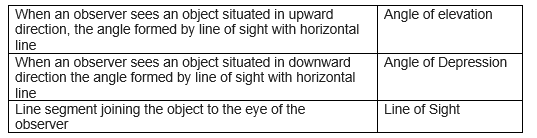Question 2.
Match the Following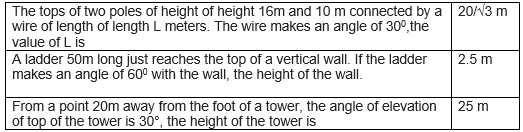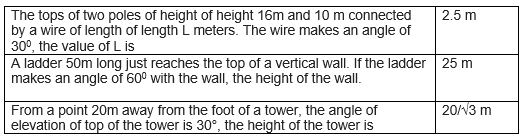## Multiple Choice Questions

Question 3
The length of Shadow of a tower on the plane ground is √3 times the height of the tower. The angle of the elevation of the sun is
(a) 300
(b) 450
(c) 600
(d) 400

Question 4
From a window (9m above ground) of a house in a street, the angles of elevation and depression of the top and foot of another house on the opposite side of the street are 300 and 600 respectively. Find the height of the opposite house and width of the street.
(a) 12m,√ m
(b) 12m,3√ 3 m
(c) 6m,3√ m
(d) 12m,3√ m

Question 5
A vertical tower stands on a horizontal plane and is surmounted by a vertical flag staff of height h m. At a point on the plane , the angles of elevation of the bottom and the top of the flag staff are α and β respectively. The height of the tower is
(a) $\frac {h tan \alpha}{tan \beta - tan \alpha}$
(b) $\frac {h tan \alpha}{tan \alpha -tan \beta}$
(c) $\frac {h tan \beta}{tan \alpha -tan \beta}$
(d) $\frac {h tan \beta}{tan \alpha + tan \beta}$

Question 6
A pole 6 m high casts a shadow $2 \sqrt{3}$ m long on the ground, then the Sun's elevation is
(a) 30°
(b) 90°
(c) 45°
(d) 60°

Question 7
If the length of the shadow of a tower is increasing, then the angle of elevation of the sun is ?
(a) increasing
(b) decreasing
(c) Remains same
(d) Can't say

Question 8
The height of a tower and the distance of the point of observation from its foot, both, are increased by 20%. The angle of elevation of its top will (a) increasing
(b) decreasing
(c) Remains same
(d) Can't say

Question 9
An observer 1.2 m tall is 48.8 m away from a chimney. The angle of elevation of the top of the chimney from her eyes is 45°. The height of the chimney is (a) 50 m (b) 48.8 m (c) 51 m (d) 52 m
Question 10
A ladder 15 metres long just reaches the top of a vertical wall. If the ladder makes an angle of 60° with the wall. The height of the wall is (a) 7.5 √ 3 m (b) 7.5 m (c) 30 √ 3 m (d) None of these

(3) (a)
(4) (b)
(5) (a)
(6) (d)
(7) (b)
(8) (c)
(9) (a)
(10) (b)

Question 11.
Two poles of equal heights are standing opposite to each other on either side of the road which is 80m wide. From a point between them on the road the angles of elevation of the top of the poles are 60°and 30°.find the height of the poles and the distances of the point from the poles.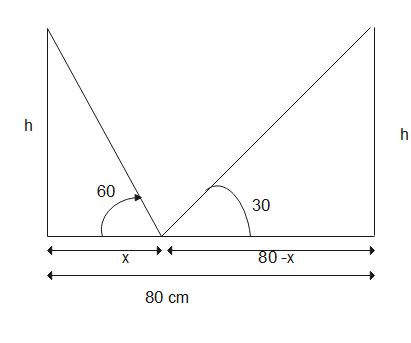Here
$tan 60 = \frac {h}{x}$
or $h= \sqrt {3} x$
Now
$tan 30 = \frac {h}{80-x}$
80 -x =3x
or x=20 m
and H= $20 \sqrt 3$ m

Question 12.
A man sitting at a height of 20m on a tall tree on a small island in the middle of the river observes two poles directly opposite to each other on the two banks of the river and in line with the foot of tree. If the angles depression of the feet of the poles from a point at which the man is sitting on the tree on either side of the river are 60° and 30° respectively. Find the width of the river.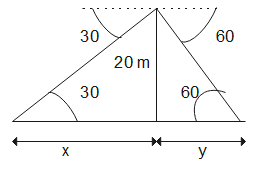Here
$tan 60 = \frac {20}{y}$
or $y= \frac {20}{\sqrt 3}$
Now
$tan 30 = \frac {h}{x}$
$x =20 \sqrt 3$
Now width of river
$d= x + y = \frac {20}{\sqrt 3} + 20 \sqrt 3= \frac {80}{\sqrt 3}$

Question 13.
A vertical tower stands on a horizontal plane and is surmounted by a flag- staff of height 7m. From a point on the plane, the angle of elevation of the bottom of the flag- staff is 30° and that of the top of the flag- staff is 45°. Find the height of the tower.

9.56m

Question 14.
A man on the deck of a ship is 10m above the water level. He observes that the angle of elevation of the top of a cliff is 45o and the angle of depression of the base is 30° calculate the distance of the cliff from the ship and the height of the cliff.

Distance = 10√3m, Height = 27.32m

Question 15.
There are two temples, one on each bank of a river, just opposite to each other. One temple is 50m high. From the top of this temple, the angle of depression of the top and the foot of the other temple are 30° and 60°respectively. Find the width of the river and the height of the other temple.

28.83m, 33.33m

Question 16.
An aero plane flying horizontally 1km above the ground is observed at an elevation of 60°. After 10 seconds, its elevation is observed to be 30°. Find the speed of the aero plane in km/hr.

415.68km/hr

Question 17.
A tree standing on a horizontal plane leaning towards east. At two points situated at distances $a$ and $b$ exactly due west on it, the angles of elevation of the top are respectively $\alpha$ and $\beta$.Prove that the height of the top from the ground is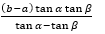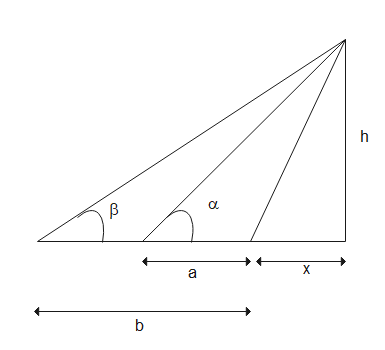Here
$tan \alpha = \frac {h}{x +a}$
or $x= \frac {h}{tan \alpha } -a$
Now
$tan \beta = \frac {h}{x +b}$
$x =\frac {h}{tan \beta } -b$
Now equating $\frac {h}{tan \alpha } -a=\frac {h}{tan \beta } -b$
$h=\frac {(b-a) tan \alpha tan \beta}{tan \alpha - tan \beta}$

## Summary

This Class 10 Maths Worksheet for Application of Trigonometry with answers is prepared keeping in mind the latest syllabus of CBSE . This has been designed in a way to improve the academic performance of the students. If you find mistakes , please do provide the feedback on the mail.You can download in PDF form also using the below linksGo back to Class 10 Main Page using below links

### Practice Question

Question 1 What is $1 - \sqrt {3}$ ?
A) Non terminating repeating
B) Non terminating non repeating
C) Terminating
D) None of the above
Question 2 The volume of the largest right circular cone that can be cut out from a cube of edge 4.2 cm is?
A) 19.4 cm3
B) 12 cm3
C) 78.6 cm3
D) 58.2 cm3
Question 3 The sum of the first three terms of an AP is 33. If the product of the first and the third term exceeds the second term by 29, the AP is ?
A) 2 ,21,11
B) 1,10,19
C) -1 ,8,17
D) 2 ,11,20